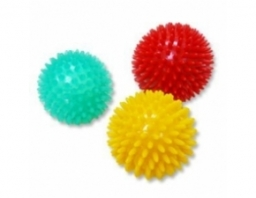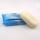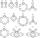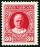# There

There are two numbers on the screen - one in the blue and the other in the red box. In the beginning, both numbers are the same. With each beep, both numbers increase - by 1 in the blue field and by 3 in the red field. At one point, the number 49 appears in the blue field and the number 129 appears in the red field. Find what numbers were initially in both boxes.

a =  9

### Step-by-step explanation:

a+1b=49
a+3b = 129

a+1•b=49
a+3•b = 129

a+b = 49
a+3b = 129

a = 9
b = 40

Our linear equations calculator calculates it.Did you find an error or inaccuracy? Feel free to write us. Thank you!Tips to related online calculators
Do you solve Diofant problems and looking for a calculator of Diofant integer equations?
Do you have a linear equation or system of equations and looking for its solution? Or do you have a quadratic equation?

## Related math problems and questions:

• Coloured numbersMussel wrote four different natural numbers with coloured markers: red, blue, green and yellow. When the red number divides by blue, it gets the green number as an incomplete proportion, and yellow represents the remainder after this division. When it div
• Diofant equation250x + 120y = 5640
• The HotelThe Holiday Hotel has the same number of rooms on each floor. Rooms are numbered with natural numerals sequentially from the first floor, no number is omitted, and each room has a different number. Three tourists arrived at the hotel. The first one was in
• ScreensThe area of a 25 inch TV screen is 300 inch² the area of a 40 inch TV screen is 768 inch². The area of the smaller screen is what percent of the area of the larger screen?
• SoapsEach box has the same number of soaps. A quarter of all boxes contain only white soaps, and in each of the remaining 120 boxes there are always half the white soaps and half the green. White soaps total 1200. (a) the number of all soap boxes; (b) the smal
• ModuloFind x in modulo equation: 47x = 4 (mod 9) Hint - read as: what number 47x divided by 9 (modulo 9) give remainder 4 .
• Two-digit numberIn a two-digit number, the number of tens is three greater than the number of units. If we multiply the original number by a number written in the same digits but in reverse order, we get product 3 478. Find the original number.
• The blueThe blue and red coats originally cost the same. Then red prices rose by 27% and blue prices fell by 87%. Then their price differed by CZK 300. How much did the originally blue coat cost?
• The rawThe raw data presented here are the scores (out of 100 marks) of a market survey regarding the acceptability of new product launched by a company for random sample of 50 respondents: 40 45 41 45 45 30 30 8 48 25 26 9 23 24 26 29 8 40 41 42 39 35 18 25 35
• Candy and boxesWe have some candy and empty boxes. When we put candies in boxes of ten, there will be 2 candies and 8 empty boxes left. When of eight, there will be 6 candies and 3 boxes left. How many candy and empty boxes left when we put candies in boxes of nine?
• Big numberhat is the remainder when dividing number 10 to 47 - 111 by number 9?
• Z9-I-4Kate thought a five-digit integer. She wrote the sum of this number and its half at the first line to the workbook. On the second line wrote a total of this number and its one fifth. On the third row, she wrote a sum of this number and its one nines. Fina
• One hundred stampsA hundred letter stamps cost a hundred crowns. Its costs are four levels - twenty tenths , one crown, two-crown and five-crown. How many are each type of stamps? How many does the problem have solutions?
• Red ballsIn the bag there are 3 red, 12 blue and 8 green balls. How many red balls we must be attached to the bag if we want the probability of pulling out the red balls was 20%?
• Diofant equationIn the set of integers (Z) solve the equation: 212x +316y =0 Write result with integer parameter t in Z (parameter t = ...-2,-1,0,1,2,3... if equation has infinitely many solutions)
• A number 5A number is divisible by 24, 25, and 120 if it is increased by 20. Find the number.
• RemaindersIt is given a set of numbers { 170; 244; 299; 333; 351; 391; 423; 644 }. Divide this numbers by number 66 and determine set of remainders. As result write sum of this remainders.